# Projectile Motion What is Projectile Motion Projectile Motion

• Slides: 21Projectile Motion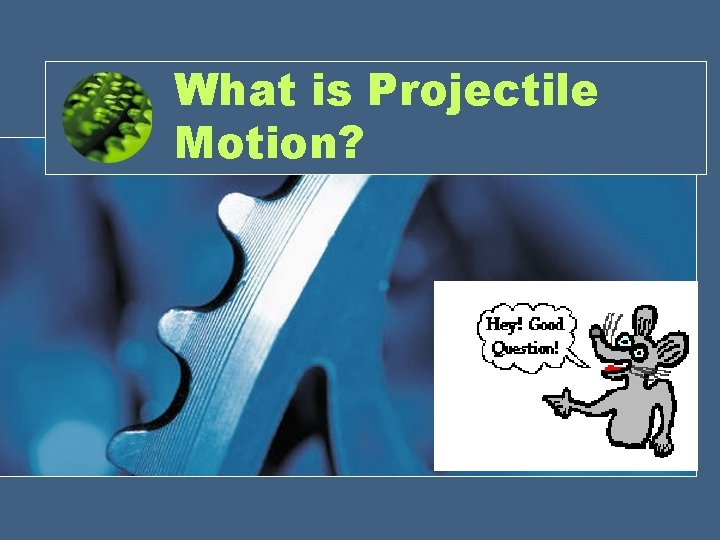What is Projectile Motion?Projectile Motion • Two-dimensional motion of an object – Vertical – HorizontalTypes of Projectile Motion • Horizontal – Motion of a ball rolling freely along a level surface – Horizontal velocity is ALWAYS constant • Vertical – Motion of a freely falling object – Force due to gravity – Vertical component of velocity changes with time • Parabolic – Path traced by an object accelerating only in the vertical direction while moving at constant horizontal velocity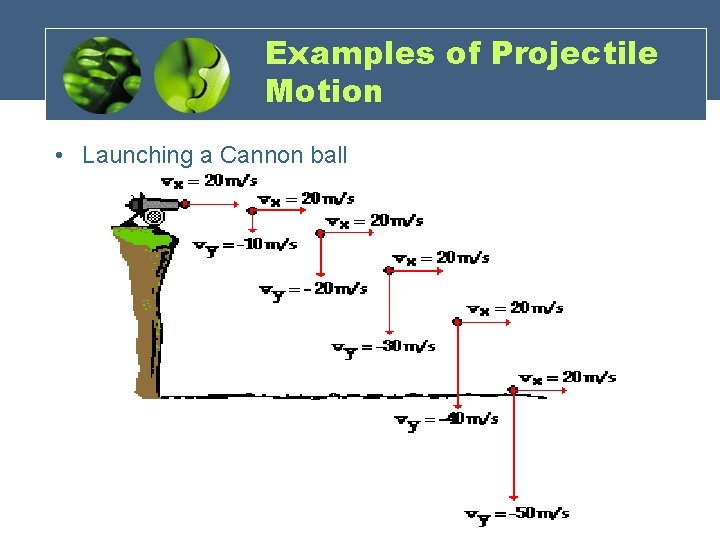Examples of Projectile Motion • Launching a Cannon ballEquations • X- Component • Y- Component Note: g= 9. 8 m/s^2 • Vectors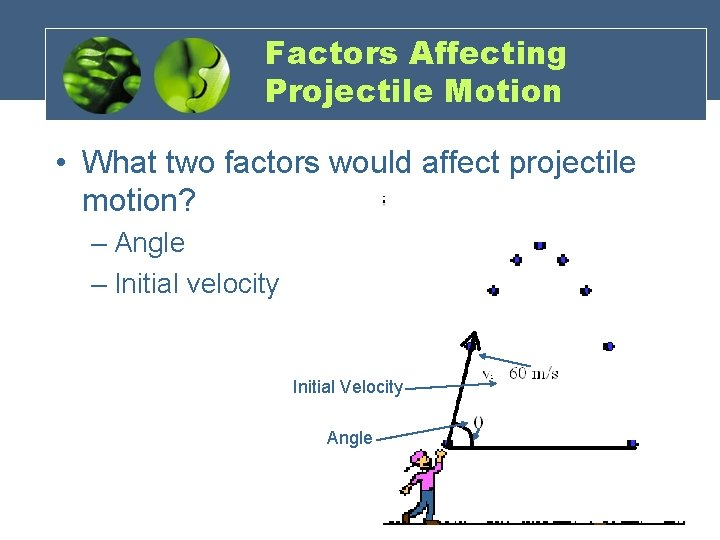Factors Affecting Projectile Motion • What two factors would affect projectile motion? – Angle – Initial velocity Initial Velocity Angle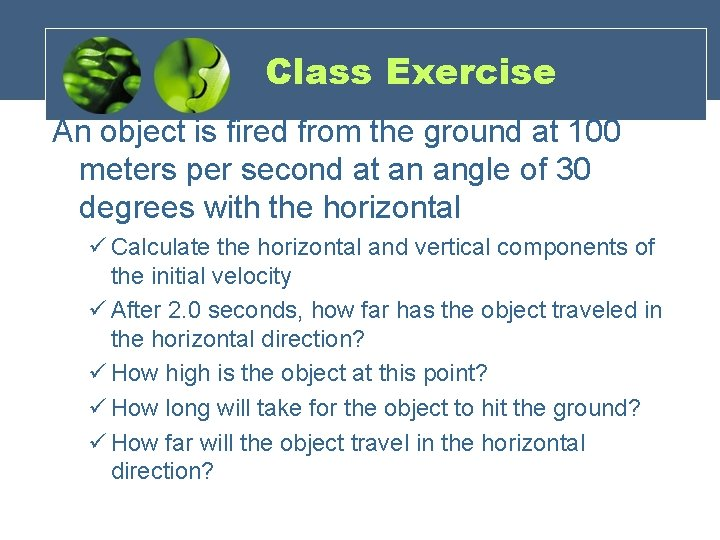Class Exercise An object is fired from the ground at 100 meters per second at an angle of 30 degrees with the horizontal ü Calculate the horizontal and vertical components of the initial velocity ü After 2. 0 seconds, how far has the object traveled in the horizontal direction? ü How high is the object at this point? ü How long will take for the object to hit the ground? ü How far will the object travel in the horizontal direction?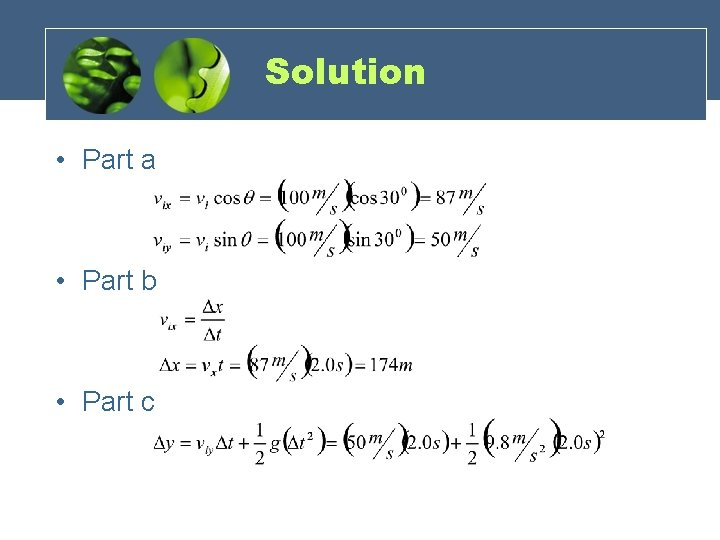Solution • Part a • Part b • Part c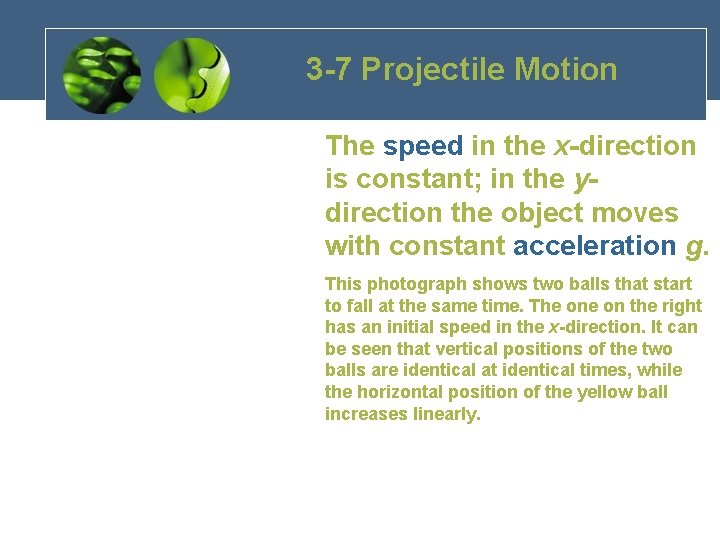3 -7 Projectile Motion The speed in the x-direction is constant; in the ydirection the object moves with constant acceleration g. This photograph shows two balls that start to fall at the same time. The on the right has an initial speed in the x-direction. It can be seen that vertical positions of the two balls are identical at identical times, while the horizontal position of the yellow ball increases linearly.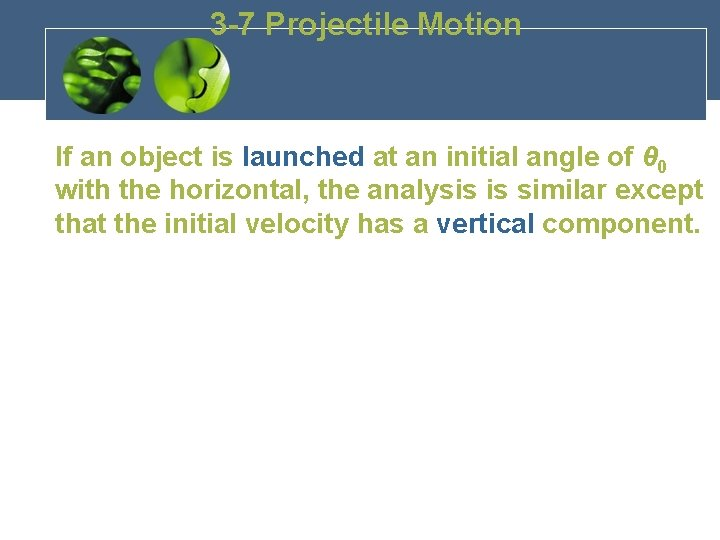3 -7 Projectile Motion If an object is launched at an initial angle of θ 0 with the horizontal, the analysis is similar except that the initial velocity has a vertical component.3 -8 Solving Problems Involving Projectile Motion Projectile motion is motion with constant acceleration in two dimensions, where the acceleration is g and is down.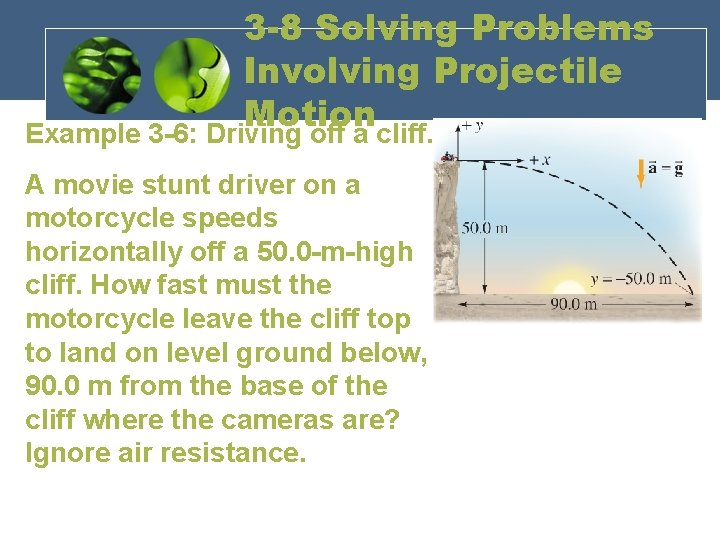3 -8 Solving Problems Involving Projectile Motion Example 3 -6: Driving off a cliff. A movie stunt driver on a motorcycle speeds horizontally off a 50. 0 -m-high cliff. How fast must the motorcycle leave the cliff top to land on level ground below, 90. 0 m from the base of the cliff where the cameras are? Ignore air resistance.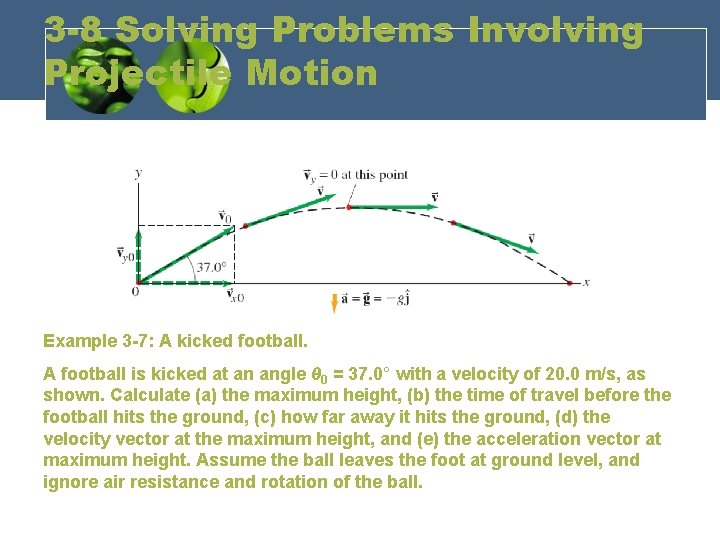3 -8 Solving Problems Involving Projectile Motion Example 3 -7: A kicked football. A football is kicked at an angle θ 0 = 37. 0° with a velocity of 20. 0 m/s, as shown. Calculate (a) the maximum height, (b) the time of travel before the football hits the ground, (c) how far away it hits the ground, (d) the velocity vector at the maximum height, and (e) the acceleration vector at maximum height. Assume the ball leaves the foot at ground level, and ignore air resistance and rotation of the ball.3 -8 Solving Problems Involving Projectile Motion Conceptual Example 3 -8: Where does the apple land? A child sits upright in a wagon which is moving to the right at constant speed as shown. The child extends her hand throws an apple straight upward (from her own point of view), while the wagon continues to travel forward at constant speed. If air resistance is neglected, will the apple land (a) behind the wagon, (b) in the wagon, or (c) in front of the wagon?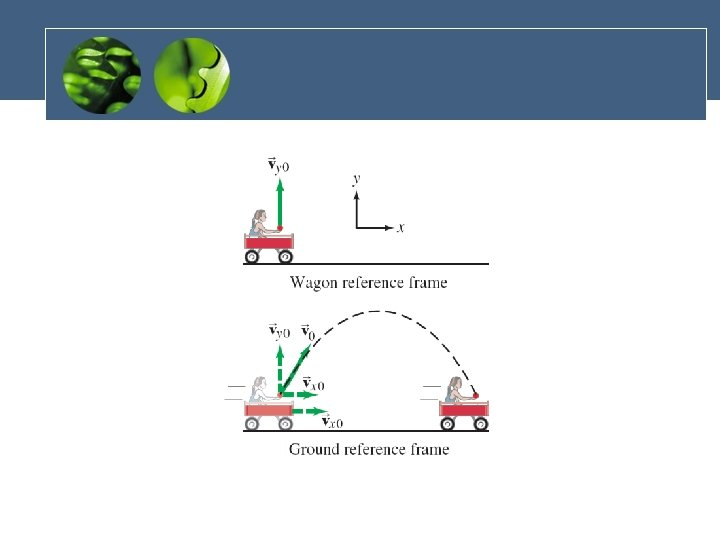3 -8 Solving Problems Involving Projectile Motion Conceptual Example 3 -9: The wrong strategy. A boy on a small hill aims his water-balloon slingshot horizontally, straight at a second boy hanging from a tree branch a distance d away. At the instant the water balloon is released, the second boy lets go and falls from the tree, hoping to avoid being hit. Show that he made the wrong move. (He hadn’t studied physics yet. ) Ignore air resistance.ApplicationsLAB TIME!!!